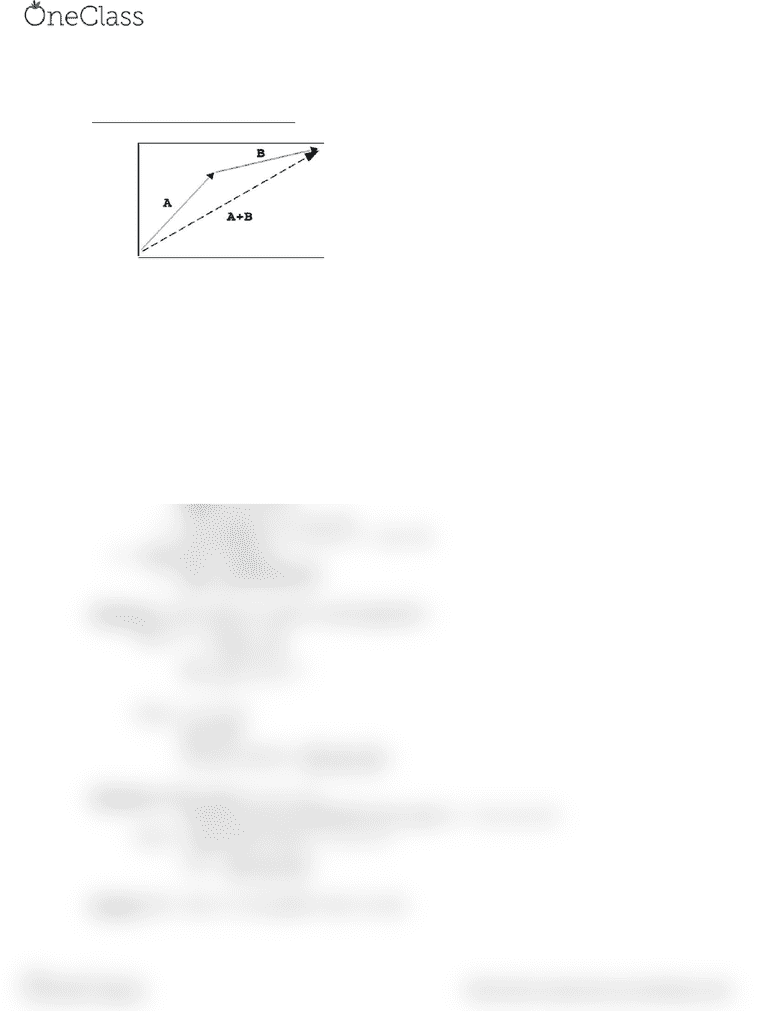# BISC 121Lg Lecture 3: Lecture 3 Notes Math

22 views2 pages
School
Professor

For unlimited access to Class Notes, a Class+ subscription is required.Lecture 3
Operations on vectors (Algebra):
Addition: use the tip to tail method
Multiplication by -1
o Flip the vector so that it goes the exact opposite way with the same starting point
Multiplication by any number (scalar), x
o If the scalar is positive:
Multiply the length of the vector by the scalar
o If the scalar is negative:
Multiply the length of the vector by the absolute value of the scalar, and
then multiply that by -1
Subtraction
o Multiply the vector being subtracted by -1 and then add that to the preexisting
vector
Algebra using coordinates:
o All vectors can be made up by the vectors i = <1, 0, 0>, j = < 0, 1 , 0>,
and k = < 0, 0, 1>
o Any vector u = xi + yj + zk
o For example: u = <1, 4, 2> = i + 4j + 2k
Magnitude of vector u:
o 
Definition: a unit vector is a vector u with magnitude 1
Ex) u = <
=
= 1
Ex) u = <-2, 1, 5>
=
The unit vector is < 


 >
Definition: If the vector u = <x, y, z>
Then the vector u multiplied by the scalar A = <Ax, Ay, Az>
Ex) A =
 and the vector u = <-2, 1, 5>
Au = < 


 >
Remark: If A = (XA, YA, ZA) and B = (XB, YB, ZB)
find more resources at oneclass.com
find more resources at oneclass.com
Unlock document

This preview shows half of the first page of the document.
Unlock all 2 pages and 3 million more documents.

Already have an account? Log in

# Get access

Grade+
\$10 USD/m
Billed \$120 USD annually
Homework Help
Class Notes
Textbook Notes
40 Verified Answers
Study Guides
1 Booster Class
Class+
\$8 USD/m
Billed \$96 USD annually
Homework Help
Class Notes
Textbook Notes
30 Verified Answers
Study Guides
1 Booster Class